Subsections

# 2.4 ArrayDeque: Fast Deque Operations Using an Array

The ArrayQueue from the previous section is a data structure for representing a sequence that allows us to efficiently add to one end of the sequence and remove from the other end. The ArrayDeque data structure allows for efficient addition and removal at both ends. This structure implements the List interface by using the same circular array technique used to represent an ArrayQueue.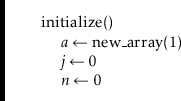The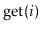and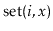operations on an ArrayDeque are straightforward. They get or set the array element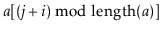.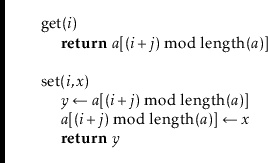The implementation of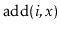is a little more interesting. As usual, we first check if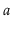is full and, if necessary, call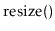to resize. Remember that we want this operation to be fast when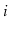is small (close to 0) or whenis large (close to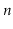). Therefore, we check if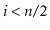. If so, we shift the elementsleft by one position. Otherwise (), we shift the elements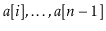right by one position. See Figure 2.3 for an illustration ofand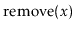operations on an ArrayDeque.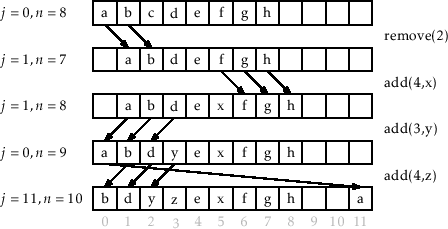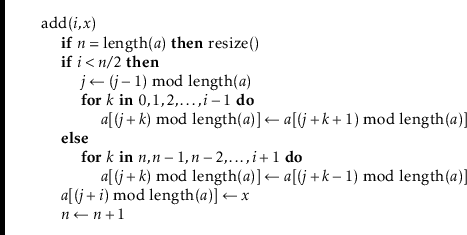By doing the shifting in this way, we guarantee thatnever has to shift more than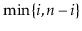elements. Thus, the running time of theoperation (ignoring the cost of aoperation) is.

The implementation of the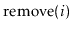operation is similar. It either shifts elementsright by one position or shifts the elements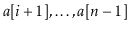left by one position depending on whether. Again, this means thatnever spends more than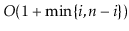time to shift elements.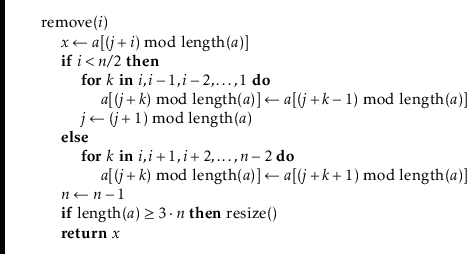## 2.4.1 Summary

The following theorem summarizes the performance of the ArrayDeque data structure:

Theorem 2..3   An ArrayDeque implements the List interface. Ignoring the cost of calls to, an ArrayDeque supports the operations
•andin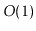time per operation; and
•andintime per operation.
Furthermore, beginning with an empty ArrayDeque, performing any sequence of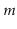andoperations results in a total oftime spent during all calls to.

opendatastructures.org